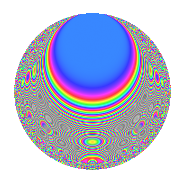# Properties

 Label 105.3.vLevel 105 Weight 3 Character orbit v Rep. character $$\chi_{105}(37,\cdot)$$ Character field $$\Q(\zeta_{12})$$ Dimension 64 Newform subspaces 1 Sturm bound 48 Trace bound 0

# Related objects

## Defining parameters

 Level: $$N$$ $$=$$ $$105 = 3 \cdot 5 \cdot 7$$ Weight: $$k$$ $$=$$ $$3$$ Character orbit: $$[\chi]$$ $$=$$ 105.v (of order $$12$$ and degree $$4$$) Character conductor: $$\operatorname{cond}(\chi)$$ $$=$$ $$35$$ Character field: $$\Q(\zeta_{12})$$ Newform subspaces: $$1$$ Sturm bound: $$48$$ Trace bound: $$0$$

## Dimensions

The following table gives the dimensions of various subspaces of $$M_{3}(105, [\chi])$$.

Total New Old
Modular forms 144 64 80
Cusp forms 112 64 48
Eisenstein series 32 0 32

## Trace form

 $$64q + 4q^{5} - 4q^{7} + 24q^{8} + O(q^{10})$$ $$64q + 4q^{5} - 4q^{7} + 24q^{8} - 16q^{10} + 16q^{11} - 48q^{15} + 80q^{16} + 56q^{17} + 24q^{21} - 96q^{22} + 72q^{23} - 4q^{25} - 288q^{26} - 380q^{28} - 48q^{30} - 136q^{31} - 48q^{32} - 72q^{33} + 76q^{35} + 384q^{36} - 28q^{37} - 68q^{38} + 164q^{40} + 128q^{41} - 12q^{42} + 344q^{43} + 240q^{46} + 412q^{47} - 288q^{48} - 72q^{50} - 24q^{51} + 388q^{52} - 40q^{53} - 8q^{55} - 864q^{56} - 192q^{57} + 56q^{58} - 180q^{60} - 216q^{61} - 912q^{62} - 84q^{63} + 20q^{65} - 72q^{66} - 368q^{67} - 492q^{68} + 416q^{70} + 784q^{71} + 36q^{72} - 316q^{73} + 96q^{75} - 32q^{76} + 844q^{77} + 624q^{78} + 908q^{80} + 288q^{81} + 556q^{82} + 1408q^{83} - 536q^{85} + 1024q^{86} + 108q^{87} + 372q^{88} + 216q^{90} - 1064q^{91} - 1704q^{92} + 144q^{93} + 260q^{95} + 352q^{97} + 272q^{98} + O(q^{100})$$

## Decomposition of $$S_{3}^{\mathrm{new}}(105, [\chi])$$ into newform subspaces

Label Dim. $$A$$ Field CM Traces $q$-expansion
$$a_2$$ $$a_3$$ $$a_5$$ $$a_7$$
105.3.v.a $$64$$ $$2.861$$ None $$0$$ $$0$$ $$4$$ $$-4$$

## Decomposition of $$S_{3}^{\mathrm{old}}(105, [\chi])$$ into lower level spaces

$$S_{3}^{\mathrm{old}}(105, [\chi]) \cong$$ $$S_{3}^{\mathrm{new}}(35, [\chi])$$$$^{\oplus 2}$$

## Hecke characteristic polynomials

There are no characteristic polynomials of Hecke operators in the database